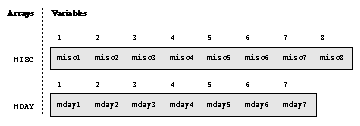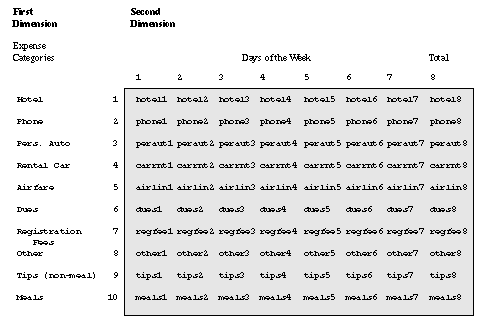Array Processing

# A Conceptual View of Arrays

The following figure is a conceptual representation of two one-dimensional arrays, MISC and MDAY.

One-Dimensional ArrayMISC contains eight elements, the variables MISC1 through MISC8. To reference the data in these variables, use the form MISC{n}, where n is the element number in the array. For example, MISC{6} is the sixth element in the array.

MDAY contains seven elements, the variables MDAY1 through MDAY7. MDAY{3} is the third element in the array.

The following figure is a conceptual representation of the two-dimensional array EXPENSES.

Example of a Two-Dimensional ArrayThe EXPENSES array contains ten groups of eight variables each. The ten groups (expense categories) comprise the first dimension of the array, and the eight variables (days of the week) comprise the second dimension. To reference the data in the array variables, use the form EXPENSES{m,n}, where m is the element number in the first dimension of the array, and n is the element number in the second dimension of the array. EXPENSES{6,4} references the value of dues for the fourth day (the variable is DUES4).Previous Page | Next Page | Top of Page